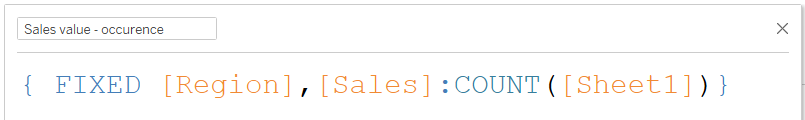top of page
Search
• Bernard K

# How to compute the mode in Tableau### Introduction

The mode is the value that appears most often in a set of data values. For example, the mode of the samples below is.

[1,2,3,4,4,4,4,4,4,4,5,7,9,10] = 4

[200,201,405,700,305,305,405,700,305,201,305] = 305

Whereby in this case, each sample has a single value as the mode (unimodal distribution). In other cases, the sample may have two modes e.g. [1,1,2,3,4,5,5,6] = 1 & 5 (a bimodal distribution), while a set with more than two modes may be described as a multimodal distribution.

In this example, I’ll be demonstrating how to compute the mode for unimodal distribution.

### The sample data

This sample data shows the sales for different product categories in four regions (East, West, Central and South). Using this data, I’m going to demonstrate how to compute the sales modal value for each region (the sales value that appears the most per region).### A quick way to compute the mode

The simplest way to compute the mode for each region is by adding Region and Sales (as a discrete value) to the rows, then add Count(Sheet1) to the labels to return the number of records per sale value per region – then sort the view in descending order by Count(Sheet1).(The sales value with the highest number of occurrences becomes the mode as highlighted in the above view)

### Proper way to compute the mode

The proper way to compute the mode, is by creating a series of calculated fields.

Whereby the first calculation will be computing the number of times each Sales value appears per region using the following FIXED LOD calculation.Next, create another calculated field computing the maximum occurrence per region - using the calculation below.Note, the above calculation could also be nested in the first calculation as shown below.Now that we’ve the number of times each sales value appears per region, and the maximum occurrence per region, we can create a Boolean calculation using these two computations to find the sales modal value using the calculation below.(The above computation returns the sales value, whenever the two calculations are equal = TRUE)

And now you can show the sales value that appears the most (sales modal value), by dragging Region to the rows and AVG ([Sales – mode]) to the text.(Note, you can use MAX () or MIN () functions to aggregate this calculation)